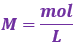# Problem: A 250.0 mL solution of 0.100 M HClO is titrated with 0.200 M NaOH. What is the expected pH of the resulting solution once 50.0 mL of the NaOH solution has been added to the HClO solution?A. 4.68B. 7.36C. 7.71D. 8.20E. 13.30

###### FREE Expert Solution
89% (472 ratings)
###### FREE Expert Solution

We are being asked to determine the pH of the HClO solution when 50.0 mL of NaOH solution is added to it.

We will calculate the pH of the HClO solution using the following steps:

Step 1. Calculate the initial amounts of HClO and NaOH in moles before the reaction happens.

Step 2. Write the chemical equation for the reaction between HClO and NaOH.

Step 3. Construct an ICF Chart.

Step 4. Calculate pH.

Step 1. Calculate the initial amounts of HClO and NaOH in moles before the reaction happens.

molarity (volume) → moles

Recall:*convert volumes from mL to L → 1 mL = 10-3 L

250 mL of 0.100 M HClO

89% (472 ratings)###### Problem Details

A 250.0 mL solution of 0.100 M HClO is titrated with 0.200 M NaOH. What is the expected pH of the resulting solution once 50.0 mL of the NaOH solution has been added to the HClO solution?

A. 4.68

B. 7.36

C. 7.71

D. 8.20

E. 13.30

What scientific concept do you need to know in order to solve this problem?

Our tutors have indicated that to solve this problem you will need to apply the Weak Acid Strong Base Titrations concept. You can view video lessons to learn Weak Acid Strong Base Titrations. Or if you need more Weak Acid Strong Base Titrations practice, you can also practice Weak Acid Strong Base Titrations practice problems.

What is the difficulty of this problem?

Our tutors rated the difficulty ofA 250.0 mL solution of 0.100 M HClO is titrated with 0.200 M...as high difficulty.

How long does this problem take to solve?

Our expert Chemistry tutor, Dasha took 8 minutes and 43 seconds to solve this problem. You can follow their steps in the video explanation above.

What professor is this problem relevant for?

Based on our data, we think this problem is relevant for Professor Tang's class at USF.